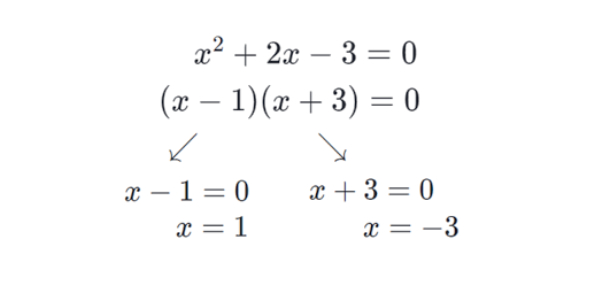# Quadratic Equations MCQ Test: Quiz!

15 Questions | Total Attempts: 5098Settings.

• 1.
The value of k for which equation  has equal roots is:
• A.

Only 3

• B.

Only -3

• C.

±3

• D.

9

• 2.
Which of the following is not a quadratic equation?
• A.
• B.
• C.
• D.
• 3.
Which of the following is solution of the quadratic equation ?
• A.

X = 2

• B.

X = -12

• C.
• D.

X = -3

• 4.
The value of k for which x = -2 is a root of the quadratic equation :
• A.

-1

• B.

-2

• C.

2

• D.

-3/2

• 5.
The value of p so that the equation  has no real root, is
• A.

P > 8

• B.

P < 5

• C.
• D.
• 6.
If px2 + 3x + q = 0 has two roots x = -1 and x = -2, the value of q – q is:
• A.

-1

• B.

1

• C.

2

• D.

-2

• 7.
The common root of the quadratic equation x2 – 3x + 2 = 0 and 2x2 – 5x + 2 = 0 is:
• A.

X = 2

• B.

X = 1

• C.

X = -2

• D.

X = 1/2

• 8.
If x2 – 5x + 1 = 0, the value of (x × 1⁄x) is:
• A.

-2

• B.

-1

• C.

1

• D.

3

• 9.
If a - 3 =  , the value of a are
• A.

5,0

• B.

5,2

• C.

-5,2

• D.

5,-2

• 10.
If the roots of the quadratic equation  the value of k is:
• A.

-1

• B.

1

• C.

2

• D.

-2

• 11.
The quadratic equation with real co-efficient whose one root is  is:
• A.
• B.
• C.
• D.
• 12.
If difference of roots of the quadratic equation x2kx + 12 = 0 is 1, the positive value of k is:
• A.

-7

• B.

7

• C.

4

• D.

8

• 13.
What is the value of k for the quadratic equation kx(x - 2) + 6 = 0 if the roots are equal?
• A.

8

• B.

-8

• C.

-6

• D.

6

• 14.
The equation  has:
• A.

Two distinct real roots

• B.

Two equal roots

• C.

No real roots

• D.

None of the above

• 15.
For an equation 2x2 – kx + 1 = 0, the roots are equal. The value of k is:
• A.
• B.
• C.
• D.
Related TopicsBack to top